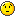# Expected limit

hello root team.

I am trying to calculate expected limit

so my code is based on twiki.cern.ch/twiki/bin/view/Ro … sMarch2015.

my code is attached.

my code shows that weird value.

In other words, my signal numbers are
37.3176
11.3302
2.34017
0.177408
0.110479
0.0282991
and background number is 34830.16
I want to get expected limit(1 sigma/2 sigma) each signal value.

can you help me?

nam
SimpleHypoTestInv.C (3.06 KB)
CountingModel.C (1.22 KB)

Hi,

You are probably scanning th values of your parameter of interest in a range too large. Try to plot the scan result and scan in a reduced region.
Attached is your modified macro scanning in [0,10]

Lorenzo
SimpleHypoTestInv.C (3.23 KB)

hello.

I used edited code, but the code also shows that weird value.

clearly, my process is like this

\$root -l

.L CountingModel.C

CountingModel(37.3176,34830.16,0.01);

.x SimpleHypoTestInv.C

I think it is no problem.

but in this case, I get

expected limit (+2 sig) 26.3158
expected limit (+1 sig) 26.3158
expected limit (median) 26.3158
expected limit (-1 sig) 26.3158
expected limit (-2 sig) 26.3158

help me.-nam

Hi,

Your scan interval is probably not correct for those model values. I have tried in the interval [25,40] and this is what I get (see also attached figure)

expected limit (+2 sig) 43.3327
expected limit (+1 sig) 38.6698
expected limit (median) 33.7233
expected limit (-1 sig) 30.4509
expected limit (-2 sig) 28.4157

Lorenzo

hello

but I still don’t know some part in code

in CountingModel.C
//my code
RooWorkspace w(“w”);
w.factory(“sum:nexp(s[10,0,135],b[10,0,34900])”); ///[color=#0000FF]nexp(s[nobs,nobs’s interval])[/color]//this is my understanding,is it something wrong?
w.factory(“Poisson:pdf(nobs[0,50],nexp)”);
w.factory(“Gaussian:constraint(b0[0,45],b,sigmab)”);
w.factory(“PROD:model(pdf,constraint)”);

//referance code
void CountingModel( int nobs = 3, // number of observed events
double b = 1, // number of background events
double sigmab = 0.2 ) // relative uncertainty in b
{
RooWorkspace w(“w”);

// make Poisson model * Gaussian constraint
w.factory(“sum:nexp(s[3,0,15],b[1,0,10])”);
// Poisson of (n | s+b)
w.factory(“Poisson:pdf(nobs[0,50],nexp)”);[color=#0000FF]how to calculate 50? what is the meaning?[/color]
w.factory(“Gaussian:constraint(b0[0,10],b,sigmab)”);
w.factory(“PROD:model(pdf,constraint)”);

I am beginner.I’m waiting your kind answer.-nam

Hi,

When defining a variable (a RooRealVar) in the workspace, for example,

``w.factory("x[ value, xmin, xmax]");``

you can provide initial value, and range, or only range. The range is used also during the minimisation to exclude regions which are unphyicals, e.g. where the pdf are not defined.